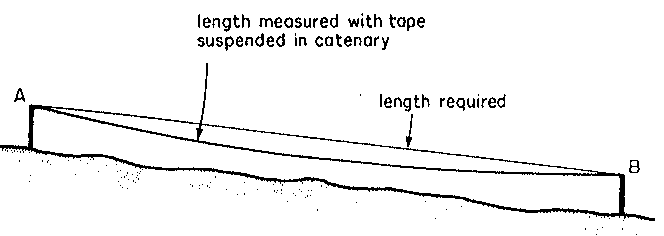Sag or Catenary Correction
When taping between two points, the tape often ends up suspended above the ground (suspended in Catenary), due to the height of the marker points. This results in the tape sagging.Correction = - w(squared) L(cubed) cos (squared)( theta) / 24 Tf (squared)

where L is the measured length and (theta) is the angle (from horizontal) of the slope between the points. Also, w is the unit weight of the tape, and Tf is the applied tension.

This value should then be added to the measured ground distance.